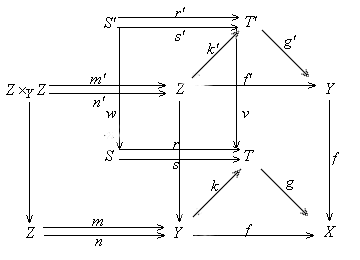5.4. Criterion for a Strict Coherent Analytic Geometrie   Generalizing the notion of an extremal epi we says that a set of maps to an object X is extremal if these maps do not factor through any given proper subobject of X.  Proposition 5.4.1. Suppose any coflat unipotent map is regular epic in a coherent analytic category A. Then A is strict.  Proof. If {ui: Ui --> X} is a finite analytic cover of an object X, then the sum of {ui} is a coflat unipotent map to X, thus is regular epi by assumption. This implies that X is the colimit of system {UiUj}. Thus  A is strict.  The following crucial result is adapted from [Diers 1992, p.93, Theorem 3.4.1].  Proposition 5.4.2. Consider a category with finite limits and finite colimits.  (a} Any coflat universal epi has a reg-epi-mono factorization.  (b} Any coflat extremal universal epi is regular.  Proof. (a) Suppose f: Y --> X is a coflat universal epi. Let k: Y --> T be the coequalizer of its kernel pair (m, n) and let g: T --> X be such that gk = f. Then k is a regular epi. First we show that {id: Y --> Y, k: Y --> T} is the fibre product of {f: Y --> X, g: T --> X}. Let f', m', n', k', g' be the images of f, m, n, k, g respectively by the pullback functor along f. The morphism f' is a split epi, therefore is a regular epi. Since the pullback functor preserving limits, thus (m', n') is the kernel pair of f'. The relation g'k' = f' implies that f' factors through k'. The relation k'm' = k'n' implies that k' factors through f'. Also f is coflat implies that k' is epic. So both f and f' are epic, which implies that k' = f', and g' is an isomorphism. Hence Y is the fibre product of f and g.Next let (r, s): S --> T be the kernel pair of f. Let r' and s' be the images of r and s respectively by the pullback functor along f, and let v, w be the maps such that gv = fg', rw = r'v, and sw = vs'. Then (r', s') is the kernel pair of g'. Because g' is isomorphism, we have r' = s', hence rw = vr' = vs' = sw. But f is universal epic implies that  w is epic. Thus r = s and hence g is monic, and (k, g) is the reg-epi-mono factorization of f.  (b) If the map f in (a) is extremal then g must be an isomorphism, so f = k is a regular epi.  Proposition 5.4.3. A coherent analytic category is strict iff any finite analytic cover is extremal.  Proof. Let {ui: Ui --> X} be a finite analytic cover of an object X. Let U be the sum of {Ui} and let u: U --> X be the map induced by the map ui. Then the coherent geometry is strict iff u is a regular epi for any analytic cover {ui}. If u is a regular epi then it is extreme, thus {Ui} is also extreme. Conversely, if the condition holds, then u is an extremal universal epi, which is coflat by (1.4.3.c), thus is regular by (5.4.2.b).  Proposition 5.4.4. A mono in a strict coherent analytic geometry is direct iff it is strong and analytic.  Proof. By (1.3.8.b) and (1.6.6.d) any direct mono is regular and analytic. Conversely, assume v: V --> X is a strong and analytic mono. By (3.1.10)V is covered by a finite set {Ui} of analytic subobjects. Thus V and {Ui} form an analytic cover on X . Since A is strict, X is the colimit of system consisting of V and {UiUj}. Since V is disjoint with each UiUj, X is the sum of V and the colimit U of the system {UiUj}. Thus V is a direct mono.   [Next Section][Content][References][Notations][Home]